# Difference Between A Function & A Relation (3 Examples)

Relations and functions are important for seeing connections between two data sets.  However, it is also important to know the key distinction between a function and a relation.

So, what is the difference between a function and a relation?  A function is a specific type of relation, where each input has only one output.  A relation may have ambiguity, since a given input can have two or more different outputs.  A function and a relation are both a set of ordered pairs (input comes first, and output comes second).

Of course, it is important to remember that every function is a relation, but not every relation is a function.  A function can also have two or more inputs that lead to the same output.

In this article, we’ll talk about the difference between functions and relations.  We’ll also look at some examples of each one and answer some common questions about them.

Let’s get started.

## What Is The Difference Between A Function & A Relation?

A function is a specific type of relation, where each input value has only one output value.  This eliminates ambiguity – that is, we always know what to expect from a function.

If we give one specific input, we know that there will only be one output.  Compare this to a relation, where one specific input can have two or more different outputs.

### Is Every Function A Relation?

Every function is a relation, since a function is a specific type of relation.  Both a function and a relation are a set of ordered pairs.

Remember that each ordered pair has two values: an input (the first value) and an output (the second value).

The diagram below will help to illustrate how functions and relations are connected.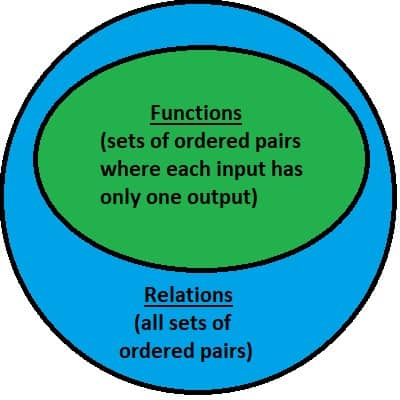This diagram shows that functions are a smaller group contained within the larger group of relations.

### Is Every Relation A Function?

Not every relation is a function.  As you can see in the diagram above, there is space in the “relations” circle that is outside of the “functions” circle.

These are the relations that are not functions.  This can be confusing, so let’s connect it to something more familiar.

A good analogy is to think of functions as dogs and relations as animals.  All dogs are animals, but not all animals are dogs (for example, there can also be cats, birds, and fish).

Likewise, all functions are relations, but not all relations are functions.

Here are some examples of relations that are not functions.

### Relations That Are Not Functions (3 Examples)

There are many relations that are not functions.  Remember that the key characteristic of a function is that each input has only one output.

So, each of these examples have an input that has two or more outputs.

#### Example 1: Height & Weight

Let’s say your x-variable (input) is height in inches, and your y-variable (output) is weight in pounds.  The ordered pairs in this relation would be a height and a weight, such as (67, 154).Height and weight is a relation, but it is not a function. Two people of the same height could have different weights.

This relation is not a function.  Think about it: how common is it for two people of the same height to have different weights?

It is quite common.  For instance, with two people who are both 57 inches tall, one could weigh 140 pounds and the other could weigh 160 pounds.

Since a given input (a height of 57 inches) can have more than one output (weights of 140 and 160 pounds), we know that this relation is not a function.

#### Example 2: Age & Income

Let’s say your x-variable (input) is age in years, and your y-variable (output) is annual income in dollars.  The ordered pairs in this relation would be an age and an income, such as (42, \$60000).Age and income is a relation, but it is not a function. Two people of the same age could earn a different salary.

This relation is not a function.  Think about it: how common is it for two people of the same age to have different incomes?

It is quite common.  For instance, with two people who are both 42 years old, one could have an income of \$40,000 per year, and the other could have an income of \$140,000 per year.

Since a given input (an age of 42 years) can have more than one output (income of \$40,000 and \$140,000), we know that this relation is not a function.

#### Example 3: Eye Color & Hair Color

Let’s say your x-variable (input) is eye color, and your y-variable (output) is hair color.  The ordered pairs in this relation would be an eye color and a hair color, such as (blue, red).Eye color and hair color is a relation, but not a function. Two people with blue eyes could have different hair colors (for example, red and blond).

This relation is not a function.  Think about it: how common is it for two people with the same eye color to have different hair colors?

It is quite common.  For instance, with two people who both have blue eyes, one could have blond hair and the other could have red hair.

Since a given input (blue eyes) can have more than one output (blond hair and red hair), we know that this relation is not a function.

### Functions That Are Not One-To-One (3 Examples)

Even if a relation is a function, the function may not be one-to-one if it has repeating y values.  A function is one-to-one if no two inputs (x-values) lead to the same output (y-value).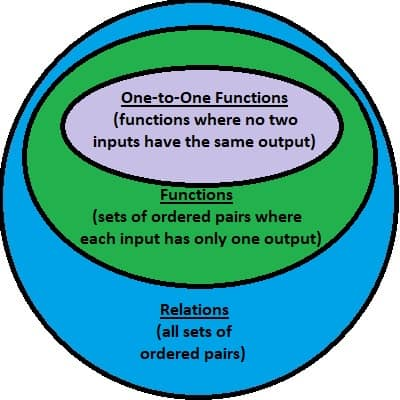This diagram shows relations (large blue circle), functions (medium green oval), and one-to-one functions (small purple oval)

Here are some examples of functions that are not one-to-one.

#### Example 1: Menu Items & Prices

Let’s say your x-variable (input) is a menu item at a restaurant, and your y-variable (output) is the price of that item.  The ordered pairs in this relation would be a menu item and a price, such as (chicken parmesan, \$25).

This relation is a function, since a given menu item (input) will have only one price (output).  Imagine how confusing it would be to get a menu where you are charged a random price for your food!

Although this is a function, it is not one-to-one.  The reason is that two different menu items (inputs) can have the same price (output).

For instance, the chicken parmesan and the broccoli alfredo could both have a price of \$25.  As ordered pairs, we would see (chicken parmesan, \$25) and (broccoli alfredo, \$25).

Since two different inputs (menu items) can have the same output (price), we know that this function is not one-to-one.

#### Example 2: Person & Age

Let’s say your x-variable (input) is a person, and your y-variable (output) is the person’s age in years.  The ordered pairs in this relation would be a person and an age in years, such as (Paul, 26).

This relation is a function, since a given person (input) will have only one age (output).  Your age may change throughout your life, but at a given moment, you only have one age!

Although this is a function, it is not one-to-one.  The reason is that two different people (inputs) can have the same age (output).

For instance, two friends (Paul and Peter) are different people, but they could have the same age.  As ordered pairs, we would see (Paul, 25) and (Peter, 25)

Since two different inputs (people) can have the same output (age), we know that this function is not one-to-one.

#### Example 3: The quadratic function y = x2

Let’s say your x-variable (input) is any real number, and your y-variable (output) is the square of that number.  The ordered pairs in this relation would be a number and its square, such as (4, 16).

This relation is a function, since a given number (input) will have only one square (output).

Although this is a function, it is not one-to-one.  The reason is that two different numbers (inputs) can have the same square (output).

For instance, the numbers 4 and -4 are different, but they have the same square.  As ordered pairs, we would see (4, 16) and (-4, 16).

Since two different inputs (real numbers) can have the same output (square), we know that this function is not one-to-one.

### Functions That Are One-To-One

As mentioned earlier, functions are a subset of relations.  Similarly, one-to-one functions are a subset of functions.

A function is one-to-one if no two inputs (x-values) lead to the same output (y-value).

Here are some examples of functions that are one-to-one.

#### Example 1: Person & Social Security Number

Let’s say your x-variable (input) is a person, and your y-variable (output) is the social security number.  The ordered pairs in this relation would be a person and a social security number, such as (Joe Smith, 012-34-5678).

This relation is a function, since a given person (input) will have only one social security number (output).

This function is also one-to-one.  The reason is that two different people (inputs) will never have the same social security number (output).

Social security numbers are meant to be a unique identifier: each person is assigned a single social security number, and each social security number is assigned to only one person.

#### Example 2: Element & Proton Count

Let’s say your x-variable (input) is an element on the periodic table, and your y-variable (output) is the number of protons that element has in its nucleus.  The ordered pairs in this relation would be an element and a number of protons, such as (Helium, 2).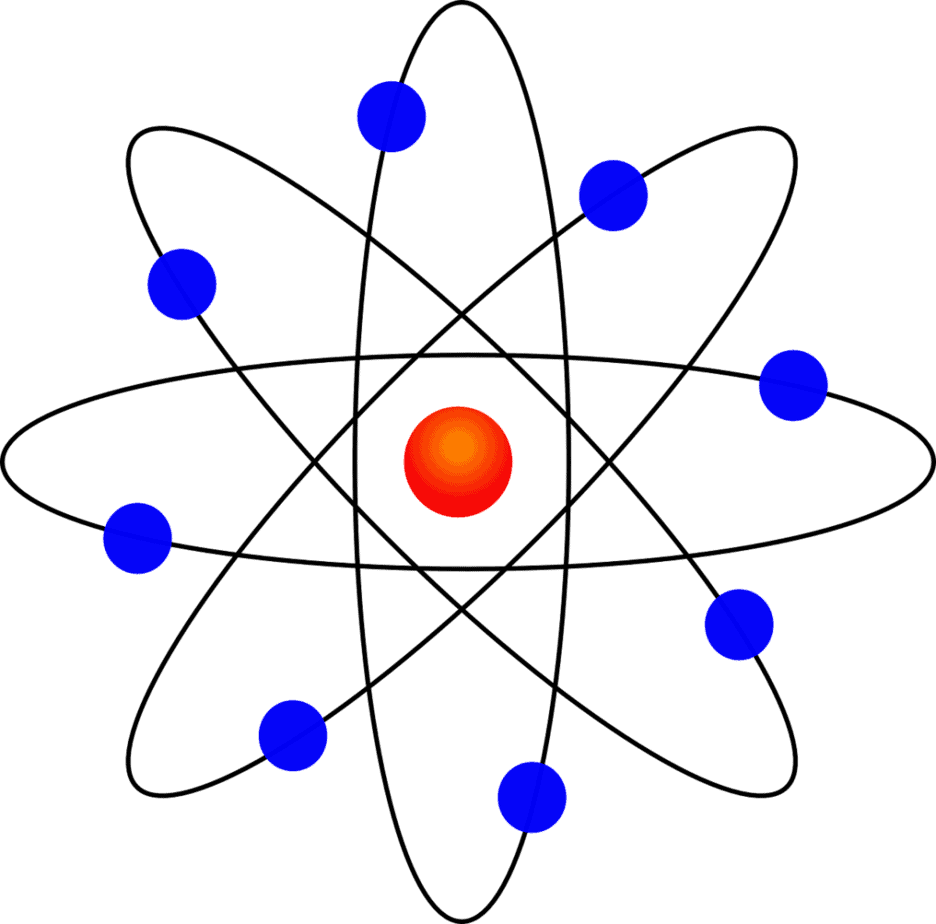Element and proton count is a one-to-one function. Each element has only one proton count, and two different elements cannot have the same proton count.

This relation is a function, since a given element (input) will have only one proton count (output).

This function is also one-to-one.  The reason is that two different elements (inputs) will never have the same proton count (output).

Note: we would have to exclude talking about ions or decaying radioactive matter for the sake of this example!

#### Example 3: Product & Item ID

Let’s say your x-variable (input) is a product in a catalog, and your y-variable (output) is the Item ID of the product.  The ordered pairs in this relation would be a product and an item ID, such as (shovel, 123456).

This relation is a function, since a given product (input) will have only one item ID (output).  This makes it easier for the company to track inventory and sales.

This function is also one-to-one.  The reason is that two products (inputs) will never have the same item ID (output).

If two products did have the same item ID, then it would be impossible to know which product a customer had ordered just based on the item ID!  This would also make it much more difficult to track inventory and sales.

## How To Identify A Function From A Graph Of A Relation (Vertical Line Test)

You can tell whether the graph of a relation is a function or not – just by looking at it!  All you need to do is learn how to use the vertical line test.

The vertical line test says that if you can draw a vertical line that goes through the graph of the relation more than once, then the relation is not a function.

Alternately, if each vertical line intersects the graph of the relation at most once, then the relation is a function.

Let’s take a look at some examples and counterexamples to solidify the concept.

### Example 1: y = 3x is a function

The graph of the relation y = 3x is pictured below: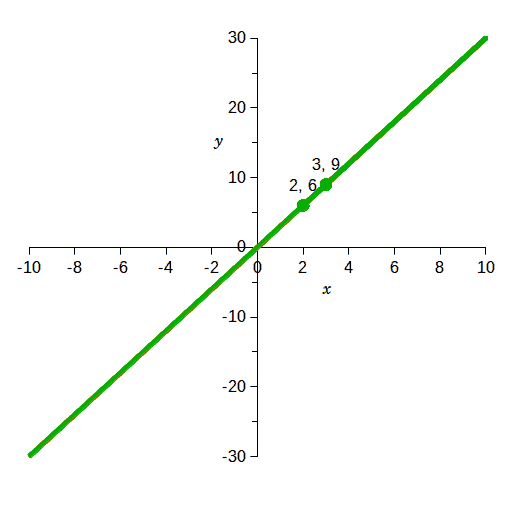The graph of y = 3x. Any vertical line intersects this relation only once, so it is in fact a function. (As it turns out, it is a one-to-one function!)

This graph represents a function, since any vertical line we draw will intersect the relation at only one point.  More specifically, a vertical line x = a will intersect the graph at y = 3a, or the ordered pair (a, 3a).

Since each vertical line intersects the relation at most once, the relation is a function.

*Note: this function is also one-to-one, since it passes the horizontal line test. It is also an odd function, since it is symmetric about the origin.

### Example 2: x2 + y2 = 1 is not a function

The graph of the relation x2 + y2 = 1 is pictured below – it is a circle with radius 1 that is centered at the origin:A circle is a relation, but not a function. If we draw a vertical line through the center of the circle, it will intersect the graph at the top and bottom (thus failing the vertical line test).

This graph does not represent a function, since we can find multiple vertical lines that intersect the relation more than once.

For example, let’s choose the vertical line x = 0, which cuts the circle into two halves (left and right).

This line will intersect the circle twice: once at (0, 1) and again at (0, -1).

Since there is a vertical line that intersects the graph more than once, this relation is not a function.

## Is A Line A Function?

Every line (except a vertical line) is a function.  As we saw earlier, the line y = 3x is a function, since each vertical line intersects it only once.

However, consider the vertical line x = 4.  This relation fails the vertical line test.

We can draw a vertical line (x = 4) that intersects the relation more than once.

Thus, any vertical line is not a function, and any non-vertical line is a function.

## Is A Quadratic A Function?

Every quadratic is a function.  However, there is no quadratic that is a one-to-one function.

A quadratic has the form y = ax2 + bx + c.  This means that for any given value of x (input), there is only one value of y (output).

However, we can find a horizontal line that intersects a parabola twice, so it is not a one-to-one function.

The horizontal line test tells us that if any horizontal line we draw will intersect a function at most once, then it is a one-to-one function.

For example, consider the parabola y = x2 + 4x – 5 (pictured below):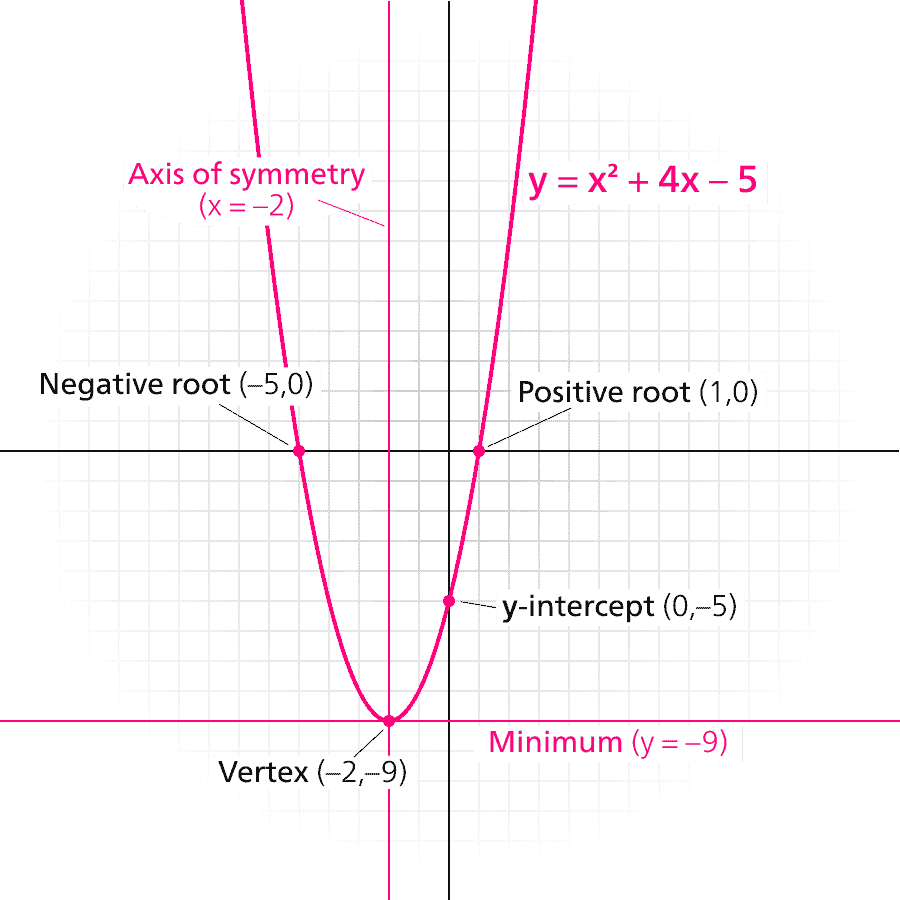The quadratic pictured above is a function, but it is not one-to-one, since it fails the horizontal line test for the line y = 0.

If we draw the horizontal line y = 0, then it will intersect the parabola at two points: (-5, 0) and (1, 0).  This means that the function fails the horizontal line test (it is not a one-to-one function).

## Is A Circle A Function?

A circle is not a function.  We can apply the vertical line test by drawing a vertical line that goes through the center of the circle.

If the center of the circle is (a, b) and the radius is R, then the vertical line x = a will intersect the circle twice: at (a, b + R) and (a, b – R).

However, the upper (or lower) half of a circle is a function.  For a circle with center (a, b) and radius R, the equation is:

(x – a)2 + (y – b)2 = R2

If we solve this equation for y, we get:

y = (R2 – (x – a)2)1/2

This equation represents the top half of the circle and it is a function.  The bottom half of the circle is also a function, and is given by

y = -(R2 – (x – a)2)1/2

## Conclusion

Now you know all about the differences between a function and a relation.  You also know how one-to-one functions fit in, and how to use the vertical line test and horizontal line test for functions.

You might also want to check out my article on even functions.